# Complex Expression Example - 6.3

## Talend Data Mapper User Guide

EnrichVersion
6.3
EnrichProdName
Talend Big Data Platform
Talend Data Fabric
Talend Data Management Platform
Talend Data Services Platform
Talend MDM Platform
Talend Real-Time Big Data Platform
Design and Development
EnrichPlatform
Talend Studio

This section shows an example of how several expressions can work together.

Example 3. Complex Expression Example

```Concat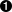Add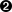First Value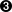Transaction-856/Segment-ISA/Element-I01
Second Value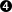Const "2"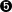Const "-"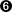LoopIndex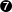Looping Output Element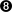Transaction-856/Loop-HL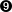```
 The root expression is a Concat function expression, which is a variable argument function expression. The first argument to the Concat function expression is an Add function expression. The second argument is an LoopIndex function expression. Both of these arguments are fixed argument functions, so each can have only one child expression. The function argument expressions for the fixed argument functions (Add and LoopIndex). Each of these can have only one child expression. A Constant function expression with the value 2 (as specified in the expression properties). This is the value of the second argument to the Add function expression. A Constant function expression with the value - (as specified in the expression properties). This is the value of the second argument to the Concat function expression. A LoopIndex function expression. This returns the index value of the Loop expression of the specified output map element. This is the value of the third argument to the Concat function expression. An output map element reference, the single argument to the LoopIndex function expression.

In this example, we want to construct a value that is a reference to an input map element plus the number 2, followed by a -, followed by the loop index of the Loop-HL output map element. So if the value of Element-I01 is 5, and we are on the tenth iteration of the Loop-HL, the value of the expression tree is 7-10.

One way to read an expression tree is from the top down. We see that the root does a Concat, at the next level down it is copying the values of the result of an Add, a Constant and an LoopIndex. We then look at each of those expressions to see how they are constructed to get their respective values.